CIM Created Edited

# [CIM/Sys. Definition] Object classification according to property information (Body / Member)

## Question

What is the difference between a body and a member?

Body represents a point, line or a plane object. A Member represents an object with both shape information and member information.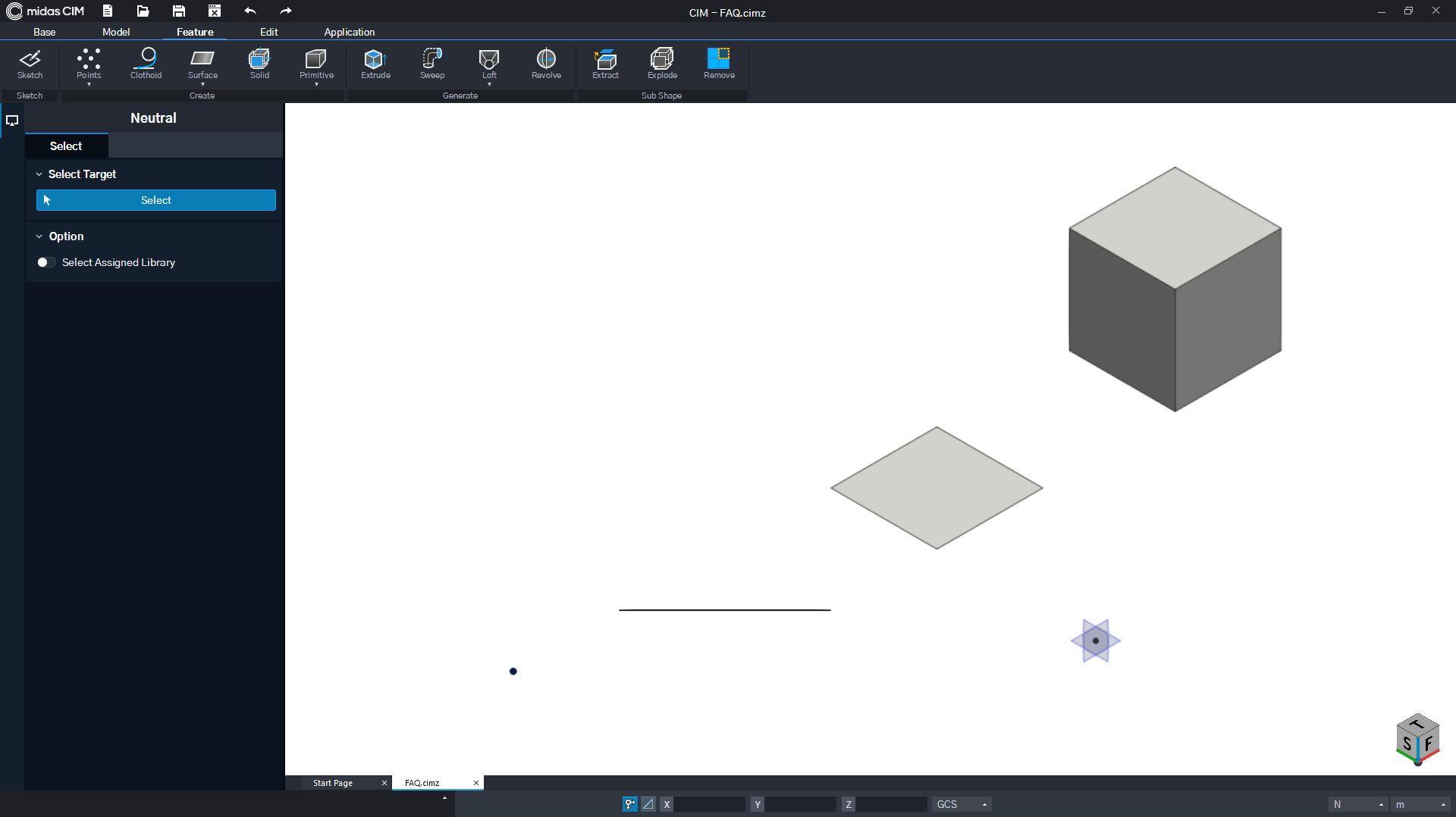Figure. 1 CIM 3D modeling environment

CIM provides a three-dimensional modeling environment.

Points, wires, surfaces, and solid elements can be freely created and each created object has its property information.

Users can use various object property information to create outputs based on CIM models such as drawings/quantities/interpretations.

## 1. What is object attribute information?

Object property information is largely divided into two categories.

Objects have a variety of property information. This property information can be divided into two main categories depending on its characteristics.

• Shape Property : Property that determines appearance, such as shape or color
Geometry / Graphic / Section (Only 1D Member) / Thickness (Only 2D Member)

• Member Property : Property of a member with material properties
Material / Analysis / Work Type / Structure

## 2. What is the absence information of an object?

By default, only objects with member properties can generate drawings / quantities / analysis results, but not all objects in the CIM model space have the member properties.

Objects are divided into Body and Member based on the availability of member properties.

All objects have feature properties.

• Body : Point, Wire, Surface, Solid object with only shape properties

• Member : Solid object with both shape and member properties
* All members are solid objects with volume

## 3. What are Body and Member?

This document talks about the concept of a body and member object.

If the user understands and utilizes the characteristics of each object well, modeling will be straightforward and easy to use for any project.

The topics below will cover the definition and explanation of the terms ‘D’ and ‘Geometry’ that are used throughout the tutorial.

D' (Dimension) in 3D space

The ‘D’ that is generally used in three-dimensional space as 0D/ 1D / 2D / 3D stands for Dimension.

Here, Dimension is referred to as the number of directions in which a point can move in the space. The following figure is an example of the above mentioned.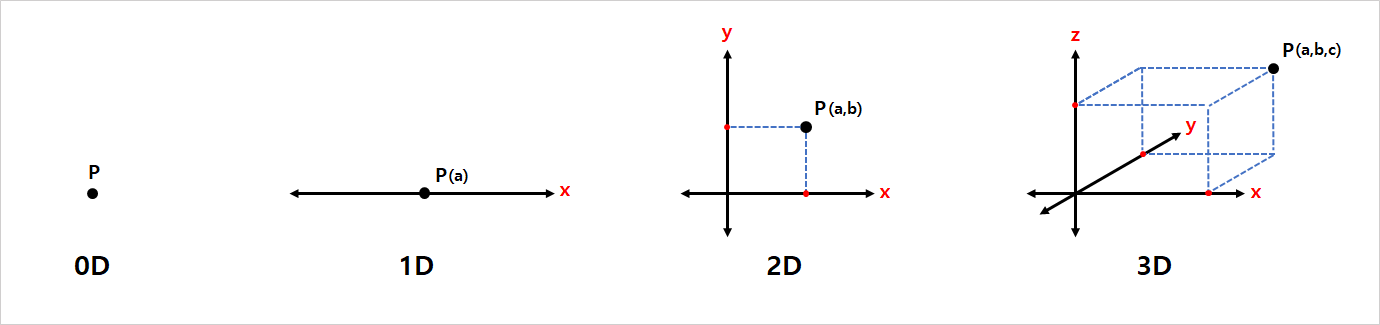This concept applies equally to Points, Wires, Surfaces, and Solid objects, and the number of directions in which any point on the object can move determines the dimension of the object.

This is a theoretical concept, and the contents are simply summarized as follows: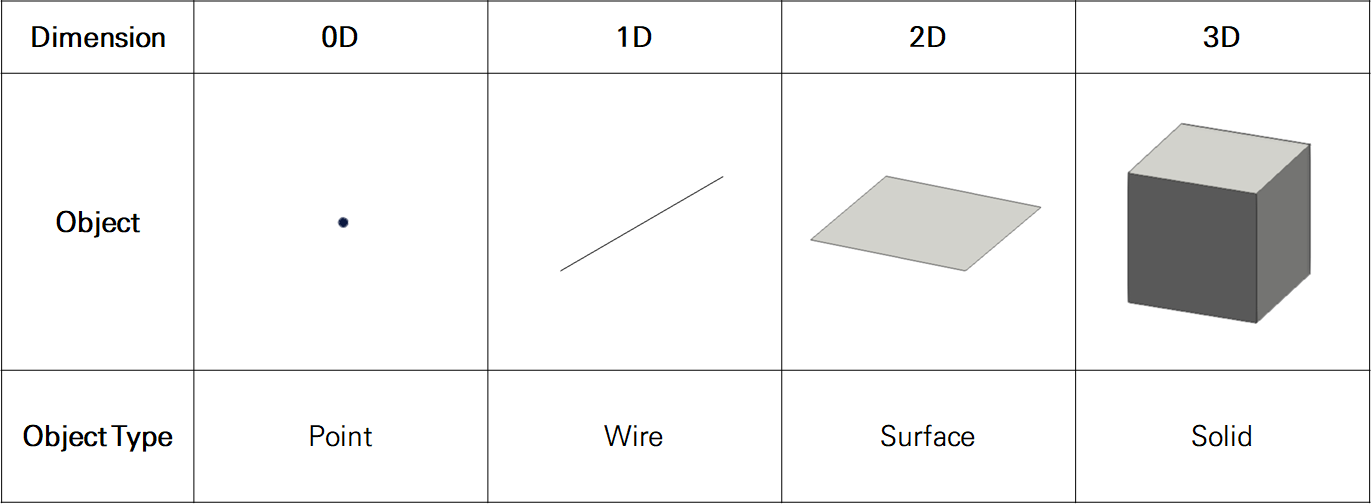'Geomerty' in modeling

In simple terms, Geometry refers to coordinate-based shape inform.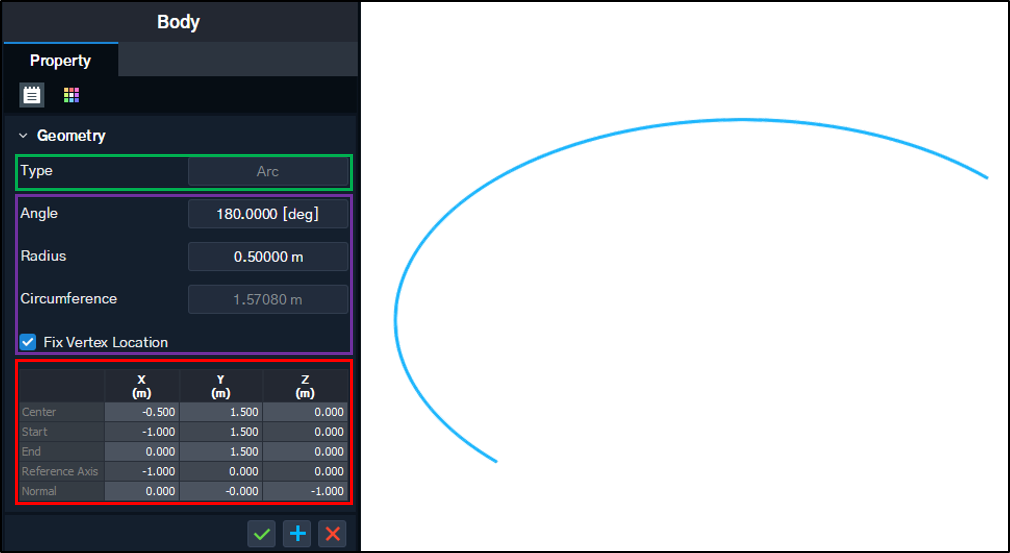The above figure is an example of selecting a line object drawn in CIM. Looking at the properties windows on the left, the user can see that the Geometry information is shown, and further see that the Arc object was drawn using the coordinate information (table) by the above rules (Angle, Radius, Circumference).

The composition may be different depending on the Geometry Type, but basically all Geometry information is composed as above.

The description of the Geometry of each object Type is as follows:

Geometry of a Point Object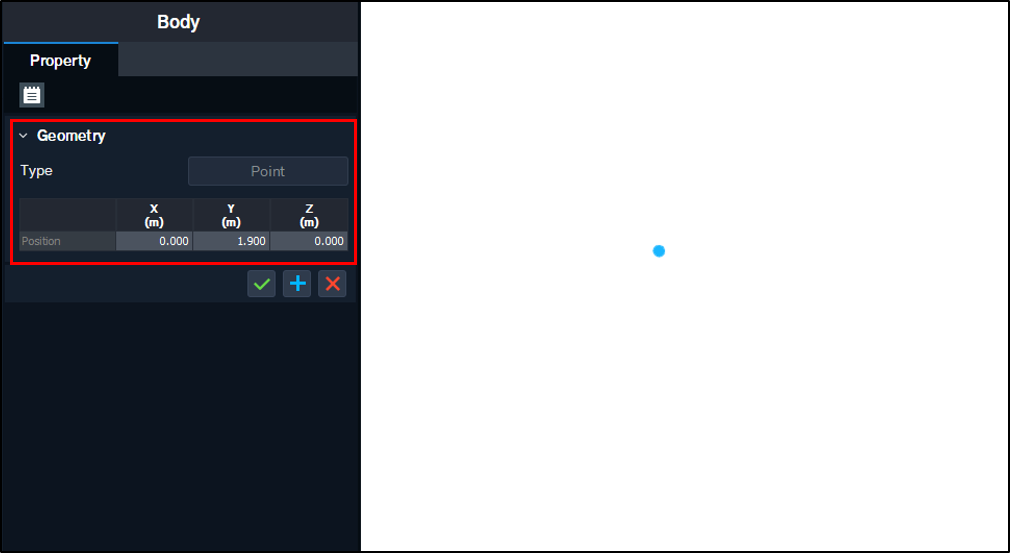The following figure shows an example of the Geometry property windows of a Point Object.
A point object has only a Point as Geometry Type, and has coordinate information of only the corresponding point.

Geometry of a Wire Object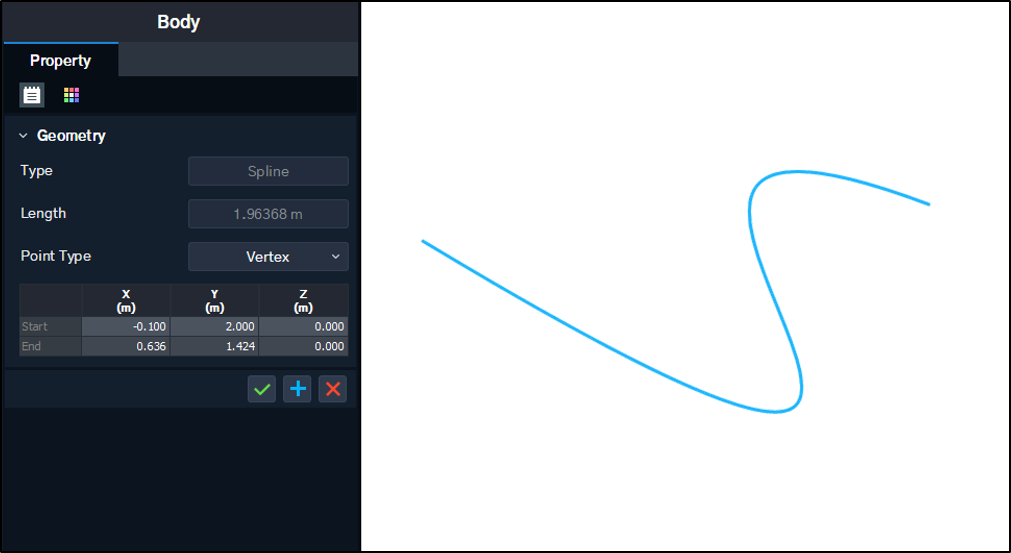The following figure shows an example of the Geometry property windows of a Spline Wire Object.
It has all Geometry Types drawn as a Wire, such as Lines, Arcs, and Splines. Furthermore, it has the coordinates necessary to draw a Wire and additional information for each type as property information.

Geometry of a Surface Object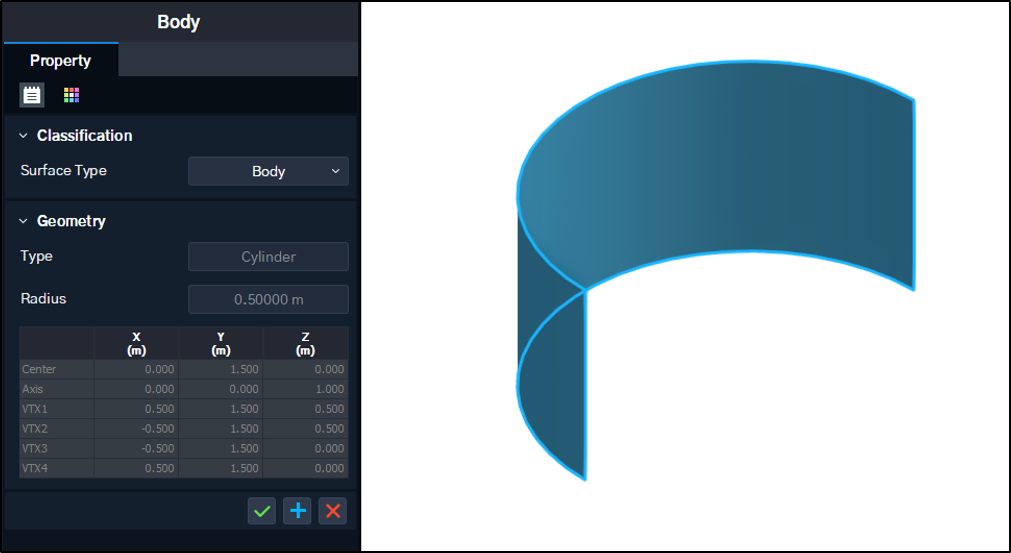The following figure shows an example of the Geometry property windows of a Cylinder Surface Object.
It has all Geometry Types drawn as a Surface, such as Planes, Cylinders, and Spheres.
Furthermore, it has the coordinates necessary to draw a Surface and additional information for each type as property information.

Geometry of a Solid Object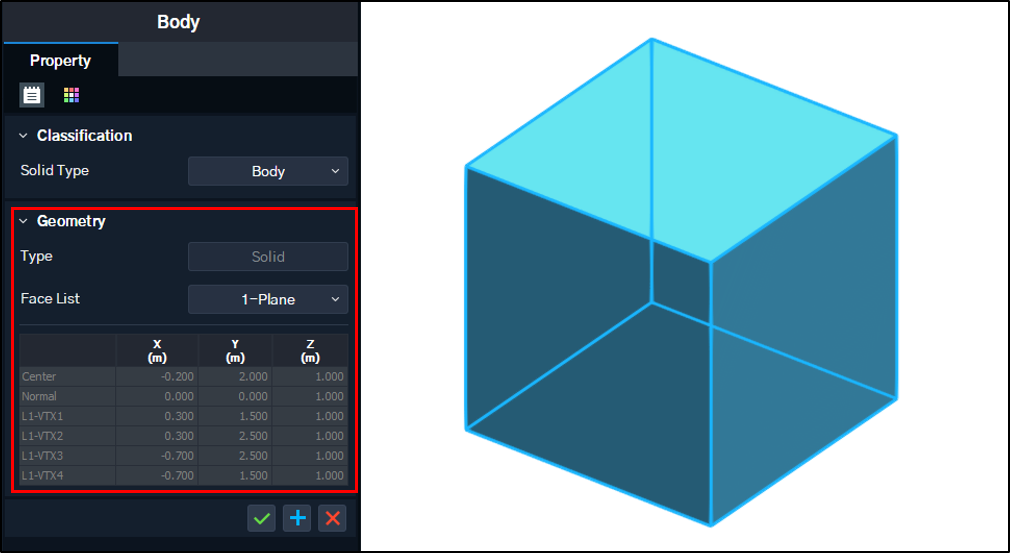Example of Geometry of a Body object among Solid Objects.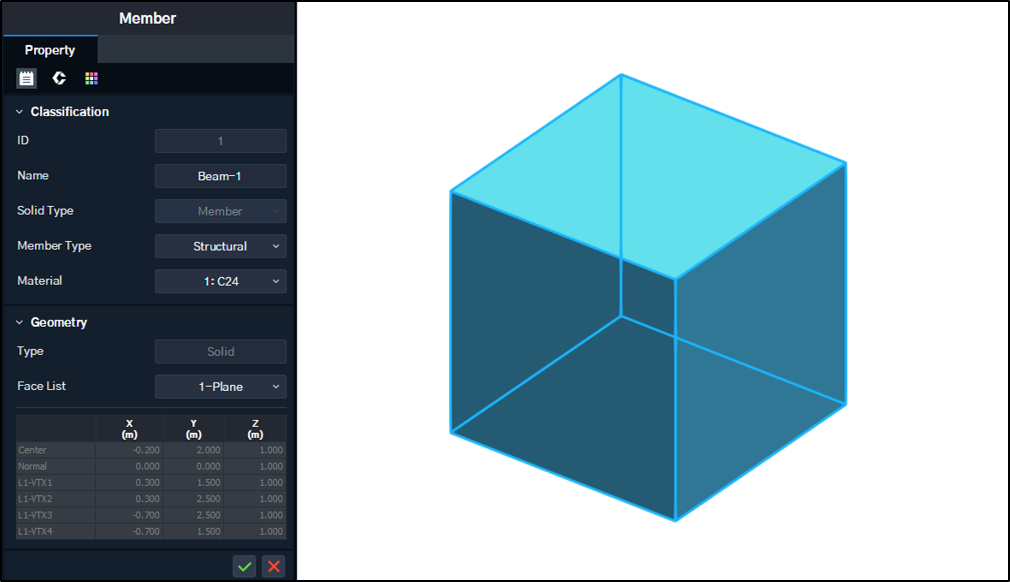Example of Geometry of a Member object among Solid Objects.
A Solid Object means any object with volume, and it is divided into Body and Member according to the property information.

• Body Object
As shown in the figure on the left, the only Geometry Type of a Body Object is a Solid.

• Member Object
In Member objects, Geometry refers to a solid-shaped skeleton, which is a three-dimensional shape, but can also have Line, Arc, Spline (1D Geometry)/ Plane (2D Geometry)/ Solid (3D Geometry) as the Geometry Type.

In the case of Member objects, the method of creating a three-dimensional shape is different depending on the geometry, and the details are covered in the following text.

## 4. Concept of a Body

A Body object refers to any object that only has shape information for its property.
(Geometry / Graphic)

A Body object does not have any additional information other than its Geometry properties that define its shape. Hence, the Geometry information of a Body is basically its shape information.

All objects, such as points, wires, surfaces, and solid, can be bodies, and are classified according to object type as shown in Figure. 2 below.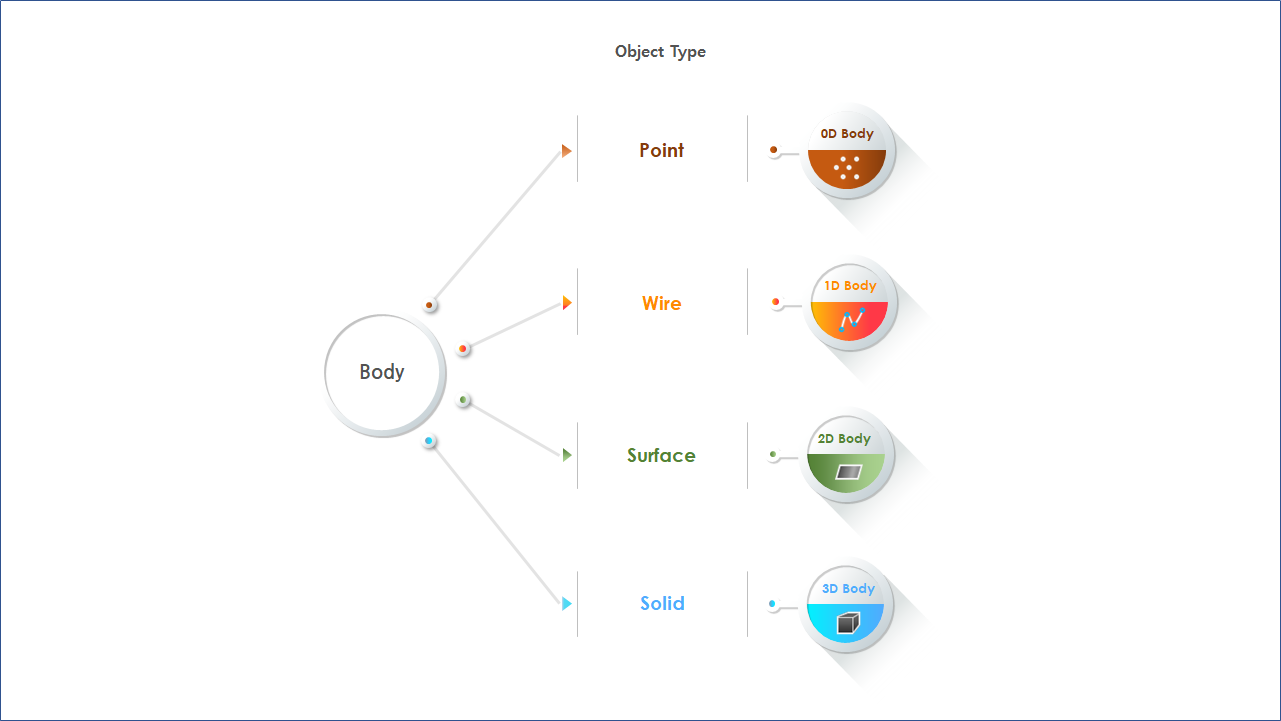Figure. 2 Body Classification

Body objects are independent from all Edit functions, so they can be utilized as 3D CAD models provided by CIM.
Hence, there is an advantage of having a high degree of freedom in shape modeling.
However, when using 3D Body (Solid Body) objects among the Body objects, the user should keep the following in mind.

Solid objects are divided into 3D Body and Member objects.
3D Body objects only have shape information, so the following needs to be kept in mind.

• Without material properties, it is not possible to generate secondary outputs such as analysis linkage, quantity calculation and drawings.

• Most functions in the ribbon menu tab model cannot be used. (Functions that require member information, such as detailed modeling of Rebar/Tendon/Stiffener, cutting functions, etc.)

## 5. Concept of a Member

A Member object refers to any object that has both shape and member information for its property.
Only solid objects can have member properties.
Hence, all members are solid objects.
By default, all member objects have member information that separate them from 3D Body objects.

Member Object Characteristics

• Quantity can be calculated using the material information.

• Rebars and tendons can be created for concrete.

• Stiffeners can be created for steel structures

• It is possible to link analyses with midas Civil.

• It is possible to link drawings with Drafter.

Member objects are divided into three categories based on how they are defined, and can be classified as shown in Figure 3 below.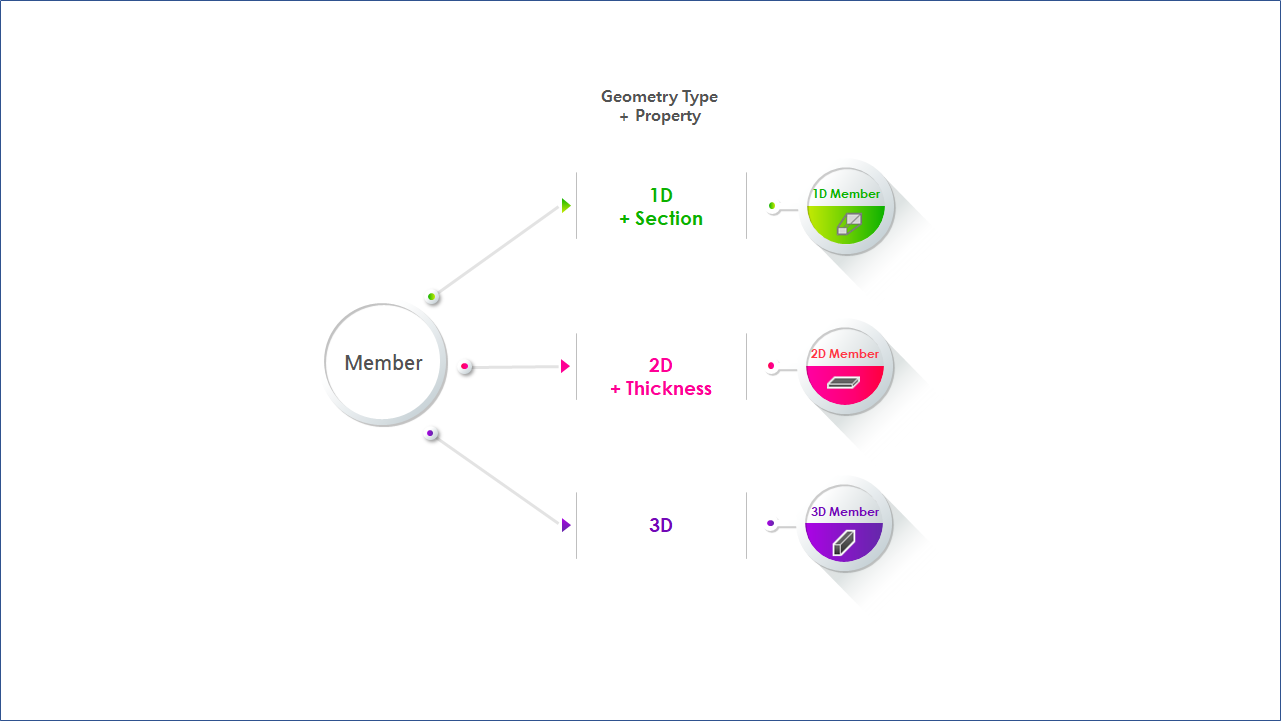Figure. 3 Member Classification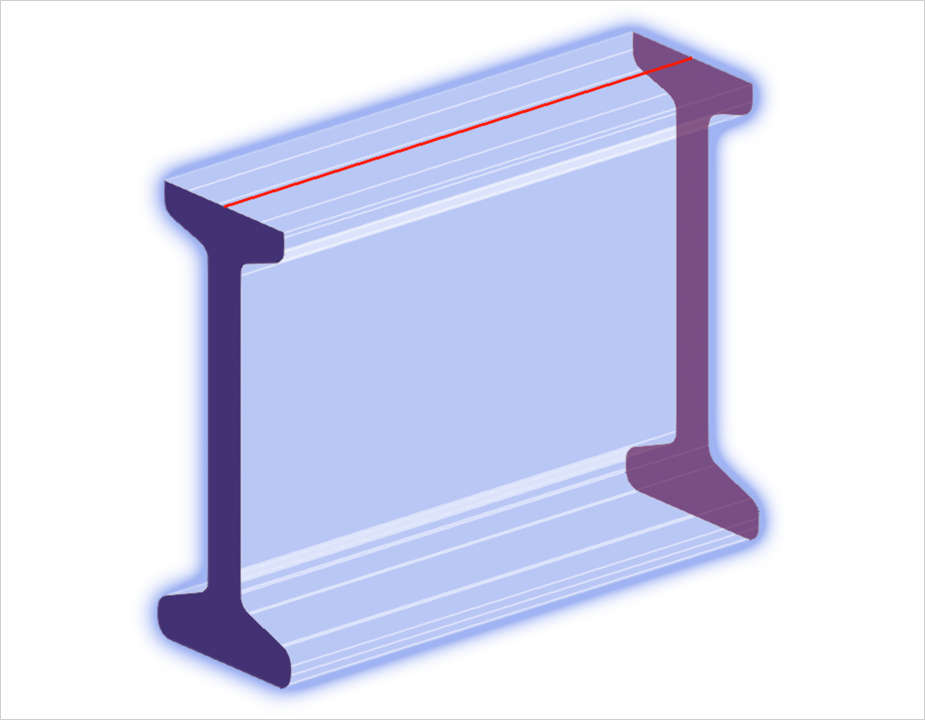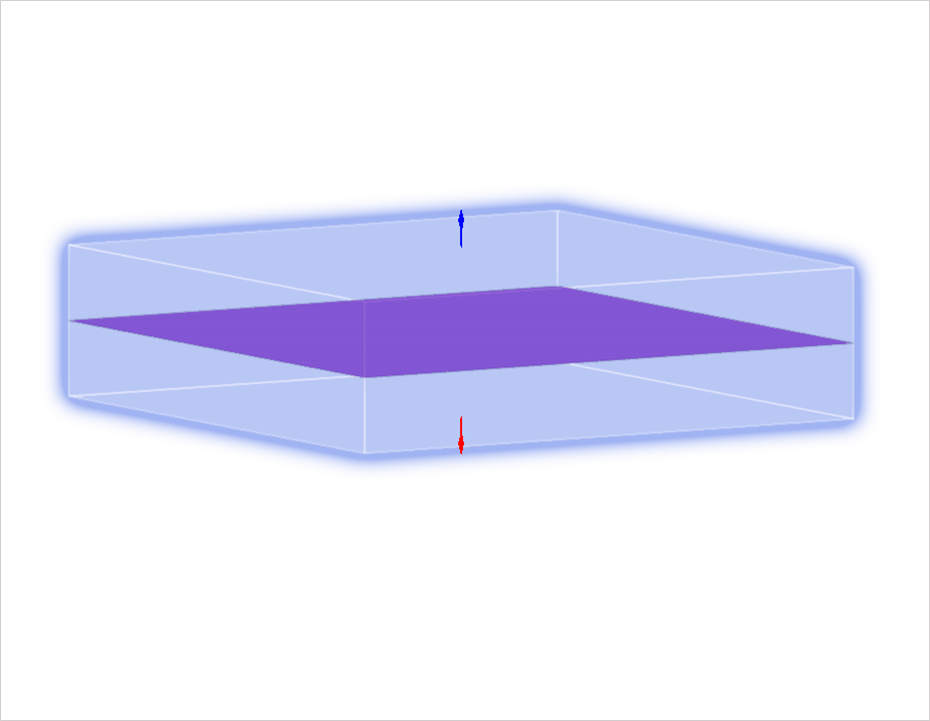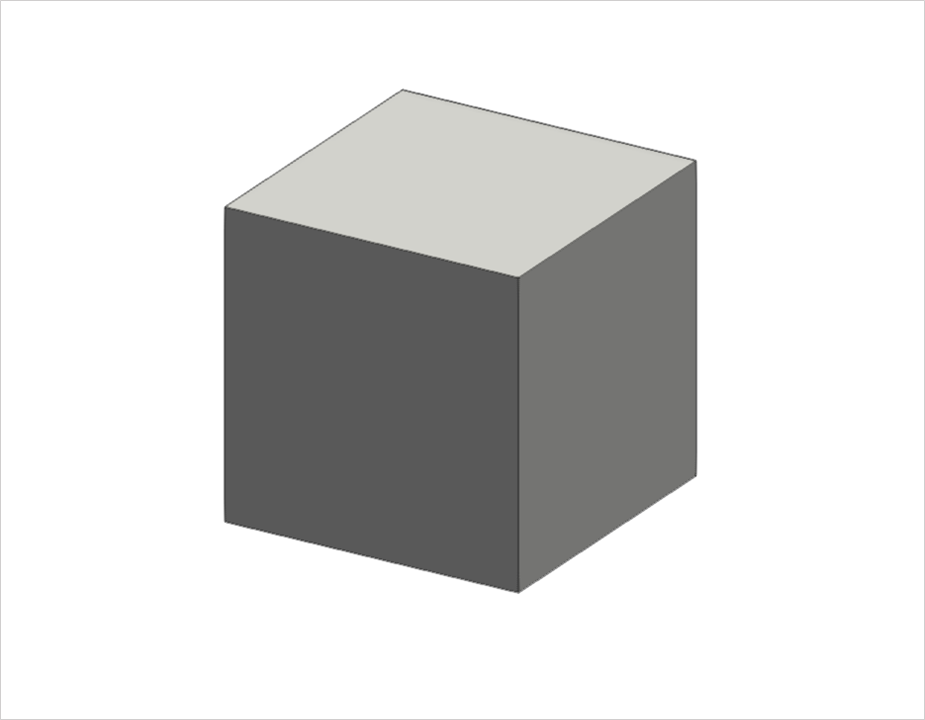Figure. 41D Member(1D Geometry with sections at the ends) Figure. 52D Member(2D Geometry with thickness) Figure. 63D Member(3D Body with Member Property)

Each member object has different usage based on how it is created.

The member object types and their respective properties are as follows

1D Member  (See Figure.4)
: Section data is applied to create a solid from a line-based 1D Geometry.

• 1D Members are critical in line-based modeling. 1D Lines can be matched with the layout to create objects that change based on the alignment information.

• 1D Line can be used to create dependent objects.
This allows objects that are created along certain paths, such as rebars and stiffeners, to change based on shape or line changes.

• Different actions can be performed based on sections. Sections can be changed based on parameters or can be replaced with superelevation or skew.

• 1D members can be linked to 1D objects and beam elements. If the section has 2D analysis data, even a 2D analysis is possible.

2D Member  (See Figure.5)
: Thickness data is applied to create a solid from a plane-based 2D Geometry.

• It is easy to model and modify based on thickness.

• This can be used for 2D analysis.

3D Member  (See Figure.6)
: Solid Geometry can be a solid by itself.

• Unlike 1D and 2D Members, it is difficult to make modifications to this model as it is not a model based on existing information such as alignment and thickness.
However, since edit and feature can be used, the shape’s degree of freedom is higher than that of 1D and 2D members.

As discussed above, bodies and members have significant differences in terms of properties and their uses.

If appropriate modeling features are used for the right purposes, the user can achieve the desired project results.

0